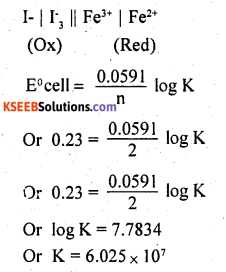# 2nd PUC Chemistry Question Bank Chapter 3 Electrochemistry

## Karnataka 2nd PUC Chemistry Question Bank Chapter 3 Electrochemistry

### 2nd PUC Chemistry Electrochemistry NCERT Textbook Questions and Answers

Question 1.
Arrange the following metals in the order in which they displace each other from the solution of their salts.
Al, Cu, Fe, Mg and Zn.
Mg, Al, Zn, Fe, Cu.

Question 2.
Given the standard electrode potentials, K+/K = -2.93V, Ag+/Ag = 0.80V,
Hg2+/Hg = 0.79 V
Mg2+/Mg = -2,37 V, Cr3+/Cr = – 0.74V
Arrange these metals in their increasing order of reducing power.
The lower the reduction potential, the higher is the reducing power. Hence, the reducing power of the given metals increases inthe following order.
Ag < Hg < Cr < Mg < K.

Question 3.
Depict the galvanic cell in which the reaction Zn(s)+2Ag+(aq) —> Zn2+(aq)+2Ag(s) takes place. Further show:
(i) Which of the electrode is negatively charged?
(ii) The carriers of the current in the cell.
(iii) Individual reaction at each electrode.
The galvanic cell in which the given reaction takes place is depicted as:
Zn(s) | Zn2+ (aq) || Ag+ (aq) | Ag(s)
(i) Zn electrode (anode) is negatively charged
(ii) Tons are carriers of current in the cell and in the external circuit, current from silver to Zinc.
(iii) The reaction taking place at the anode is given by,
Zn(s) -H → Zn2+(aq) + 2e
The reaction taking place at the cathode is given
Ag++ e → Ag(s)Question 4.
Calculate the standard cell potentials of galvanic cell in which the following reactions take place:
(i) 2Cr(s) + 3Cd2+(aq) → 2Cr3+(aq) + 3Cd
(ii) Fe2+(aq) + Ag+(aq) → Fe3+(aq) + Ag(s)
Calculate the ArG9and equilibrium constant of the reactions.
(i) For the given reaction, the Nemst equation can be given as: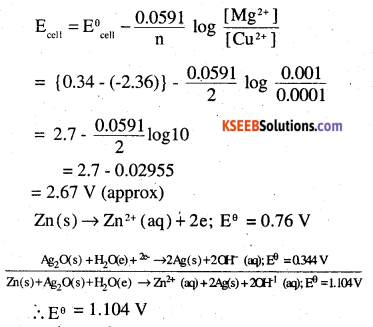∴ Eθ = 1.104V
We know that,
ΔrGθ = -nFEθ
= -2 × 96487× 1.04
= – 213043.296J
= -213.04KJ

Question 5.
Write the Nernst equation and em! of the
following cells at 298 K:
(I) Mg(s)|Mg+2(O.OO1M) ||Cu+2(0.0001M)|Cu(s) .
(ii) Fe(s)|Fe+2(O.OO1 M)||H+(1M)H2(g) (1bar)|Pt(s)
(iii) Sn(s) |Sn2+(O.050 M)||H+(0.020M|H2(g) (1 bar)|Pt(s)
(iv) Pt(s)|Br2(l)|Br(O.O1O M)||H+(O.030 M)| H2(g) (1 bar)|Pt(s).
(i) EθCr3+ /Cr = 0.74V
EθCd2+ / Cd = – 0.40V
The galvanic cell of the reaction IC depicted as :
Cr(s)|Cr3+ (aq) || (Cd2+ (aq) Cd(s)
Now, the standard cell potential is
Eθcell = EθR– EθL
= -40 – (-0.74)
= +0.34V
rGθ = —nFEθcell
In the given equation,
n = 6
F = 96487 C mol-1
Eθcell = + 0.34V
Then, ∆rGθ =-6 × 96487 mol-1 × 0.34V
= -196833.48 CV mol-1
= -196833.48 J mol-1
= -196.83 KJ mol-1
Again
rGθ = -RT In K
=>∆rGθ =-2.303RT log K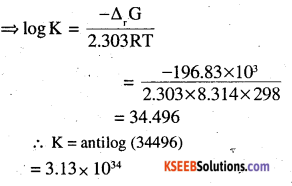(ii) Eθ Fe3+ /Fe2+ = 0.77V
Eθ Ag/Ag = 0.80V
(ii) For the given reaction, the Nernst equation can be given as: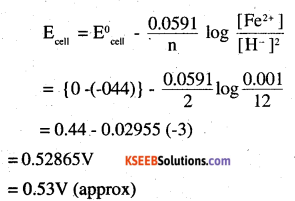(iii) Far the givën reaction, the Nernst equation can be given as: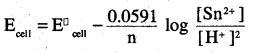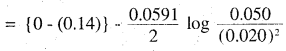0.14 – 0.0295 × log 125
= 0.14-0.062
= 0.078 V
= 0.08 V (approx)

(iv) For the given reaction , the nernst equation can be given as: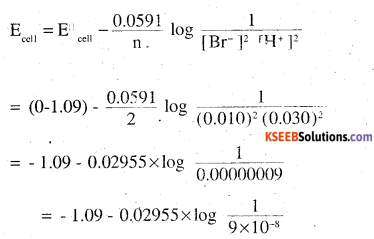= – 1.09 – 0.02955 × log ( 1.11× 107)
= – 1.09 – 0.02955(0.0453 + 7)
= -1.09 – 0.208 =-1.298 V.Question 6.
In the button cells widely used in watches and other devices the following reaction takes place:
Zn(s) + Ag2O(s) + H2O(l) → Zn2 + (aq) + 2Ag(s) + 2OH-(aq)
Determine ∆r Gθ and Eθ for the reaction.
The galvanic cell of the reaction is depicted as:
Fe2+ (aq) | Fe3+ (aq) || Ag+ (aq) | Ag(s)
Now, the standard cell potential is
Eθcell = EθR – Elθ
= 0.80 – 0.77 ‘
= 0.03 V
Here, n = 1
Then, ∆r Gθ = – nFEθcell
= -1 × 96487 C mol-1 × 0.03V
= – 2894.61 J mol-1
= – 2.89 KJ mol-1
r Gθ = 2.303 RT In K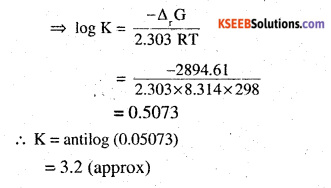Question 7.
Define conductivity and molar conductivity for the solution of an electrolyte.
Discuss their variation with concentration.
Conductivity of a solution is defined as the conductance of a solution 1 cm in length and area of cross section cm2.1 is represented by K.

Conducti vity always decreases with a decrease in concentration both for weak and strong electrolytes. This is because the number of ions per unit volume that carry the current in a solution decreases with a decrease in concentration.

Molar conductivity of a solution at a given concentration is the conductance of volume V of a solution containing 1 mole of the electrolyte kept between two electrodes with the area area of cross-section A and distance of unit length.

Molar conductivity increases with a decrease in concentration. This is because the total volume of the solution containing one mole of the electrolyte increases on dilution.

Question 8.
The conductivity of 0.20 M solution of KCl at 298 K is 0.0248 S cm-1. Calculate its molar conductivity.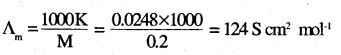Question 9.
The resistance of a conductivity cell containing 0.001M KCl solution at 298 K is 1500Ω. What is the cell constant if conductivity of 0.001M KCl solution at 298 K is 0.146 × 10-3 S cm-1.
Cell constant = conductivity × Resistance
= 0.146 × 10-3S Cm-1 × 1500 Ω = 0.219 cm-1Question 10.
The conductivity of sodium chloride at 298 K has been determined at different concentrations and thfe results are given below: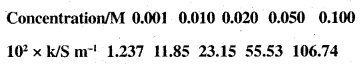Calculate ∆m for all concentrations and draw a plot between ∆m and c1/2. Find the value of ∆0m
K = 7.896 × 10-5 S cm-1
M = 0.00241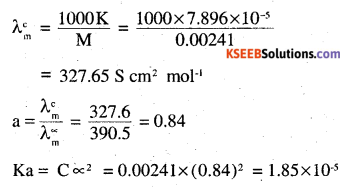Question 11.
How much charge is required for the following reductions?
(i) 1 mol of Al3+ to Al
(ii) 1 mol of Cu2+ to Cu
(iii) 1 mol of MnO4- to Mn2+
Al3+ + 3e → A1
charge required = 3F
(ii) Cu2+ + 2e → Cu
charge required = 2F
(iii) MnO4- + 8H+ + Se → Mn2+ + H2O
charge required = 5F

Question 12.
How much electricity in terms of Faraday ¡s required to produce
(j) 20.Ogat Ca from molten CaCl2
(ii) 40.0 g of Al from Almólten Al2O3
(i) Ca2+2 + 2e → Ca
2F can produce I mole (=40 g) Ca
∴ To produce 20 g Ca requires, $$\frac{2 \mathrm{F} \times 20}{40}$$ = 1F

(ii) Al3+ + 3e → Al
3F can produce 1 mole (= 27g) Al
∴ To produce 40 g A1 requires
$$\frac{3 \mathrm{F} \times 40}{27}$$ = 4.44F

Question 13.
How much electricity is required in coulomb for the oxidation of
(i) 1 mol of H2O to O2
(ii) 1 mol of FeO to Fe2O3.
(i) 2H2O → 4H+ + O2 + 4e
2F of electricity is required for oxidation of 1 mole of H2O
(ii) Fe2+ → Fe3+ + e
IF of electricity is required for oxidation of 1 mole FeO

Question 14.
A solution of Ni(NO3)2 is electrolysed between platinum electrodes using a current of 5 amperes for 20 minutes. What mass of Ni is deposited at the cathode?
Ni2+ + 2e → Ni
2F (2 × 96500 C) can produce 58.7 g of Ni
Q = It = 5 × 20 × 60 =6000C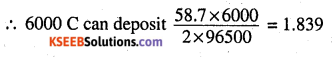Question 15.
Three electrolytic cells A,B,C containing = 0.439 g of Zn solutions of ZnSO4, AgNO3 and CuSO4, respectively are connected in series. A steady current of 1.5 amperes was passed through them until 1.45 g of silver deposited at the cathode of cell B. How long did the current flow? What mass of copper and zinc were deposited?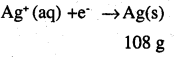i.e. 108 g of Ag is deposited by 96487 C
Therefore, 1 .45g of Ag is deposited by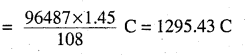Given,
Current = 1.5A
$$\frac{1295.43}{1.5}$$ S
∴ Time = 863.6S
= 864 S
= 14.40 min
Again,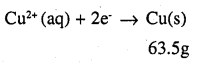i.e. 2 × 96487 C of charge deposit = 63.5 g of Cu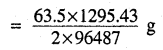Therefore, 1295.43 C of charge will deposit = 0.426g of Cu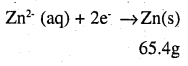i.e. 2 × 96487 C of charge deposit = 65.4 g of Zn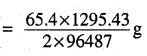Therefore, 1295.43 C of charge will deposit = 0.439 g of ZnQuestion 16.
Predict the products of electrolysis in
each of the following:
(1) An aqueous solution of AgNO3 with silver electrodes.
(ii) An aqueous solution of AgNO3 with platinum electrodes.
(iii) A dilute solution of H2SO4 with platinum electrodes.
(iv) An aqueous solution of CuCl2 with platinum electrodes.
(i) At cathode:
The following reduction reactions compete to take place at the cathode
Ag+(aq) + e → Ag(s); Eθ = 0.80 V
H+ (aq) + e → $$\frac { 1 }{ 2 }$$ H2 (g); Eθ = 0.00V
The reaction with a higher value of Eθ takes place of the cathode. Therefore, deposition of silver will take place at the cathode.

At anode:
The Ag anode is attacked by NO3 ions. Therefore, the silver electrode at the anode dissolves in the solution to from Ag+.

(ii) At cathode: Same as above
At anode: Anode is not attackable and hence OH ions have lower discharge potential than NO3 ions and OH ions react to give O2
OH → OH + e
4OH → 2H2O + O2 (g)
(iii) H2SO4 → 2H+ + SO2-4
HO2 ⇌ H+ + OH

At cathode:
2H++ 2e → H2
At anode: 4OH → 2H2O + O2 + 4e
i. e., H2 will be liberated at cathode and O2 at anode.

(iv) CuCl2 → Cu2++2Cl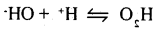At Cathode: Cu2+ ions will be reduced in preference to H+ ions
Cu2+ + 2e → Cu
At anode: Cl’ ions will be oxidised in preference to OH ions.
2Cl → Cl2 + 2e
i.e., Cu will be deposited on the cathode and
Cl2 will be liherated at the anode.

Question 1.
A solution of sodium chloride is a better
conductor of electricity at a temperature of 50°C than at room temperature. Why?
A solution of NaCl shows greater conduction of electricity at a temperature of 50°C than at room temperature because the ionic mobility of a strong electrolyte such as NaCl increases with an increase in temperature.

Question 2.
Give the relationship between molar conductivity and specific conductivity.
Molar conductivity and specific conductivity are related to each other by the given equation.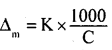Where,
Δm = Molar conductivity
K = Specific conductivity
C = Molar concentration

Question 3.
Why is it not possible to measure single electrode potential?
The process of oxidation or reduction cannot take place alone. However, electrode potential is a relative tendency and can be measured with respect to a reference electrode such as standard hydrogen electrode.

Question 4.
Why is the rusting of iron faster in saline water than in pure water?
Strong electrolytes such as sodium chloride are present in saline water. The ions produced from NaCl help in the reduction of oxygen to form water. Hence, the rusting of iron is faster in saline water than in pure water.

Question 5.
What happens Δ0m for weak electrolytes obtained by using Kohlrausch law if the migration of ions is increased to three
Δ0m for weak electrolytes obtained by using Kohlrausch law is independent of the migration of ions.Question 6.
Define molar conductivity of a solution.
The molar conductivity of a solution at a given concentration is the conductance of volume ‘V’ of a solution containing 1 mole of _ the electrolytic kept between two electrodes with cross sectional area ‘A’ and distance of unit length.
Or,
Δm = $$\frac{\Delta}{1}$$ K
Now,
1 = 1 and Δ = V (volume containing 1 mole of the electrolyte)
∴ Δm = KV

Question 7.
What are the factors that affect the conductivity of an ionic (electrolytic) solution?
The conductivity of an ionic (electrolytic) solution depends upon the following factors.

• Temperature
• Concentration of electrolyte
• Nature of the electrolyte added
• Nature of solvent and its viscosity
• Size of the ions produced and their solvation.

Question 8.
The electrolysis of a salt solution of a metal was carried out by passing a current of 4A for 45 minutes. This resulted in the deposition of 2.977g of the metal. If the atomic mass of the metal is 106.4 g mol-1, then calculate the charge present in the metal cation.
Let the charge on the metal cation be h i.e. the metal cation is M+
Accordingly,
Mh+ + 4e → M
Therefore, a current of h × 96500 coulomb will deposit 106.4 g of metal quantity of charge passed = 10800 coulombs
Now, 10800 coulombs will deposit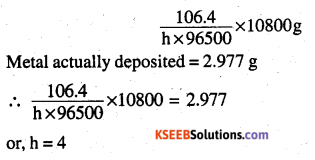Hence, the charge present on the metal cation is + 4.

Question 9.
(a) At infinite dilution, the ionic conductance of Ba2+ and Cl is 121 and 76 ohm1 cm respectively. What will be the equivalent
conductance of BaCl2 (in ohm-1 cm2) at infinite dilution?
(b) What effect does concentration have on the molar conductivity of a strong electrolyte?
(a) The molar conductivity of barium chloride is given by the following equation:
Δ0m(BaCl2) = Δ0Ba2+ + 2 Δ0Cl-
= 127 + 2 × 76 = 279Ω-1 cm2 mol-1
Δ0 eq = $$\frac{279}{2}$$ Ω-1cm2Eq-1
= 139.5 Ω-1cm2 Eq-1
[∴ Eq.wt. of BaCl2 = $$\frac{1}{2}$$ × mol.wt]
Hence the equivalent conductance of BaCl2 at infinite dilution is 139.5 Ω-1cm2 eq-1

(b) The molar conductivity of a strong electrolyte decreases with the square root of concentration in linear fashion, as shown below.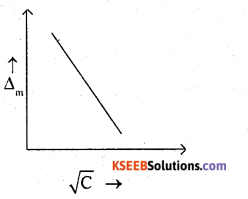Question 10.
(a) The standard reduction potentials of Fe3+ | Fe2+ and I3 | Iare 0.77 V and 0.54 V respectively for the reaction 2Fe3+ + 3I ⇌ 2Fe2+ + I3. Calculate the value of equilibrium constant.
(b) How much charge is required 1 mole of Cu2+ to Cu